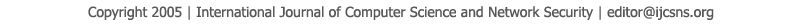To search, Click below search items.All Published Papers Search Service
 Title Author Year Keyword
 Title Minimization of Linear Constraints in Constant Slope Hybrid Dynamic Systems Author Yamen El Touati Citation Vol. 18  No. 10  pp. 19-23 Abstract Dynamic hybrid systems (DHS) cover a wide variety of real time system as well as embedded systems where the behavior is captured by an interaction between discrete and continuous components. Typically, these systems are modelled by general Hybrid Automata (HA) which extend Finite state automata by differential equations and linear inequalities on the variables that model the system. The reachability problem is known undecidable for general Hybrid Automata. This means that there is no algorithm capable to sole the general form of the problem by a computer. However, many restrictions on general hybrid automata are proposed in literature where the reachability problem becomes decidable and covers in the same time a large category of systems. We focus on an interesting class of Rectangular HA (RHA) covering important aspects of real time systems. Besides, configurations and transitions of this modelling framework are defined by conjunction of linear constraints. And, the system of constraints related to system variables forms a convex polyhedron. In many cases, the constraints are computed by specific algorithms that may produce redundant constraints and where this redundancy is not easy to disclose. In this paper, we propose an approach to transform the initial linear constraints by an equivalent and minimized polyhedron. Keywords linear constraints, polyhedron, dynamic hybrid systems. URL http://paper.ijcsns.org/07_book/201810/20181004.pdf